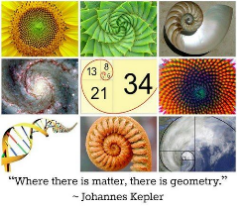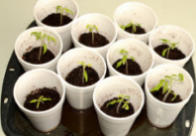# Learn at Home: Screen-Free

## Learn at Home: Screen-Free

Science and Nature:Plant Exploration

Count clusters of sprouts observed on a lawn or a local park and observe that same cluster over time.

• Measure the sprouts each day or every other day, record and graph their growth. Illustrate the daily changes as the sprout grows into a plant and flowers.

If able to get seeds, start growing seedlings at home and again measure and graph their growth. Conduct experiments where some seedlings grow:

1.  in total darkness
2. by a window and
3. in a corner.

Fibonacci ExplorationIn 1202, Italian mathematician Leonardo Pisano (also known as Fibonacci, meaning "son of Bonacci") pondered the question: Given optimal conditions, how many pairs of rabbits can be produced from a single pair of rabbits in one year? This thought experiment dictates that the female rabbits always give birth to pairs, and each pair consists of one male and one female.

­Think about it -- two newborn rabbits are placed in a fenced-in yard and left to, well, breed like rabbits. Rabbits can't reproduce until they a­re at least one month old, so for the first month, only one pair remains. At the end of the second month, the female gives birth, leaving two pairs of rabbits. When month three rolls around, the original pair of rabbits produce yet another pair of newborns while their earlier offspring grow to adulthood. This leaves three pairs of rabbit, two of which will give birth to two more pairs the following month.

The order goes as follows: 1, 1, 2, 3, 5, 8, 13, 21, 34, 55, 89, 144 and on to infinity. Each number is the sum of the previous two. This series of numbers is known as the Fibonacci numbers or the Fibonacci sequence. The ratio between the numbers (1.618034) is frequently called the golden ratio or golden number.

Activity:

Seed heads, pinecones, fruits and vegetables: Look at the array of seeds in the center of a sunflower and you'll notice what looks like spiral patterns curving left and right. Amazingly, if you count these spirals, your total will be a Fibonacci number. Divide the spirals into those pointed left and right and you'll get two consecutive Fibonacci numbers. You can decipher spiral patterns in pinecones, pineapples and cauliflower that also reflect the Fibonacci sequence in this manner. ­

Flowers and branches: Some plants express the Fibonacci sequence in their growth points, the places where tree branches form or split. One trunk grows until it produces a branch, resulting in two growth points. The main trunk then produces another branch, resulting in three growth points. Then the trunk and the first branch produce two more growth points, bringing the total to five. This pattern continues, following the Fibonacci numbers. Additionally, if you count the number of petals on a flower, you'll often find the total to be one of the numbers in the Fibonacci sequence. For example, lilies and irises have three petals, buttercups and wild roses have five, delphiniums have eight petals and so on.

The human body: Take a good look at yourself in the mirror. You'll notice that most of your body parts follow the numbers one, two, three and five. You have one nose, two eyes, three segments to each limb and five fingers on each hand. The proportions and measurements of the human body can also be divided up in terms of the golden ratio. DNA molecules follow this sequence, measuring 34 angstroms long and 21 angstroms wide for each full cycle of the double helix

Gr. 2-3 Plant ExperimentActivity:Math and Art

Pythagorean Theorem